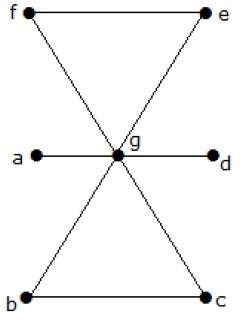# Finding the line covering number of a graph

## Problem Statement

What is the line covering number for the following graph?## Solution

Number of vertices = |V| = n = 7

Line covering number = (α1) ≥ ⌈ n / 2 ⌉ = 3

α1 ≥ 3

By using 3 edges, we can cover all the vertices.

Hence, the line covering number is 3.